1. 清华大学 汽车安全与节能国家重点实验室, 北京 100084;
2. 北京电动车辆协同创新中心, 北京 100081

Powertrain design of hydraulic hybrid track-type bull dozers based on planetary gear sets
QIN Zhaobo1, LUO Yugong1,2, ZHANG Donghao1, CHEN Long1, LI Keqiang11. State Key Laboratory of Automotive Safety and Energy, Tsinghua University, Beijing 100084, China;
2. Collaborative Innovation Centre for Electric Vehicles, Beijing 100081, China
Abstract: A powertrain design based on planetary gear sets is developed to improve the transmission efficiency of hydraulic hybrid track-type vehicles. An automated modelling framework is built based on the planetary transmission characteristics to characterize the powertrain dynamics. Screening based on the physical property requirements limits the number of feasible designs. The straight driving and steering characteristics of these feasible designs are calculated to select the better designs compared with existing designs. Finally, a dynamic programming analysis of the typical driving cycle of track-type bull dozers is used to calculate the fuel economy. Simulations show that this powertrain gives better traction, steering performance and fuel economy than existing hydraulic track-type bull dozers.
Key words: track-type bull dozer     hydraulic hybrid     planetary gear set     dynamic programming

1 基于行星传动的液压混合动力履带推土机传动系统结构

 履带推土机 运行质量/kg 9 049 履带接地长/m 2.349 履带接地宽/m 1.550 末级传动比 52.13 发动机 排量/L 4.6 额定转速/(r·min-1) 1 400 额定功率/kW 78 液压泵/马达 排量/cm3 36.4 怠速最高流量/L 80 最高转速/(r·min-1) 3 200 最高功率/kW 54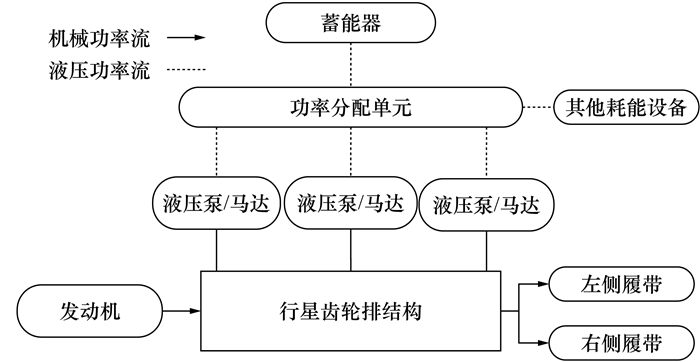图 1 液压混合动力履带推土机传动系统结构

2 系统的构型设计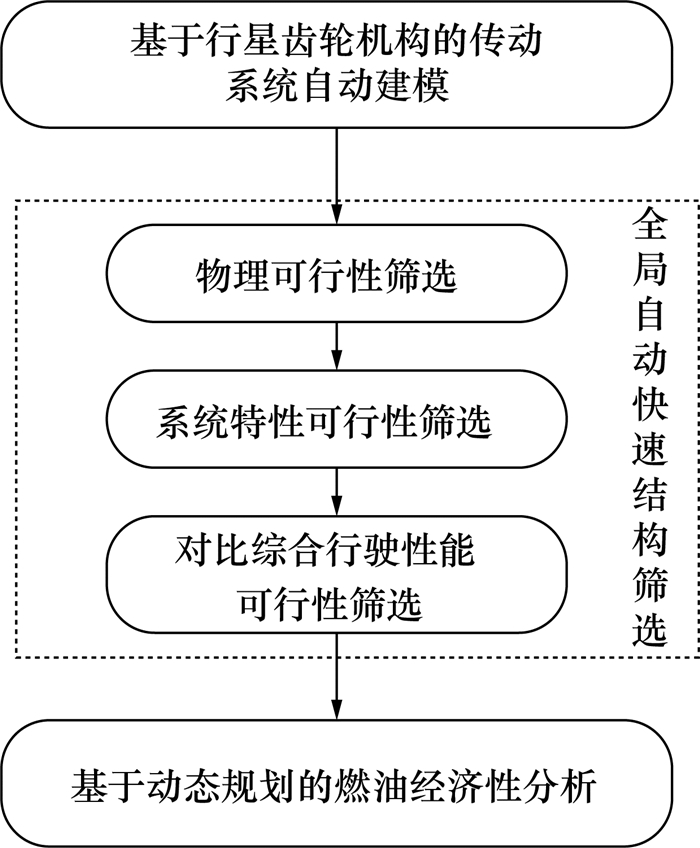图 2 系统的构型设计步骤

2.1 自动建模 2.1.1 第一动力学特征矩阵的建立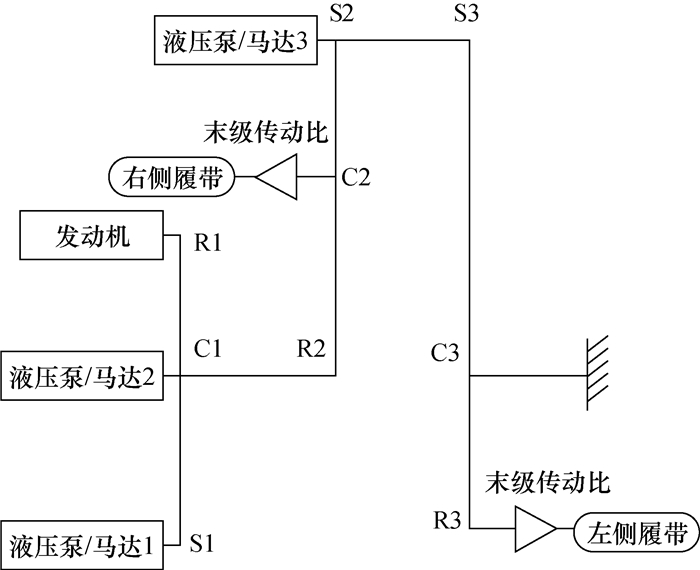图 3 一种基于3排行星传动的候选结构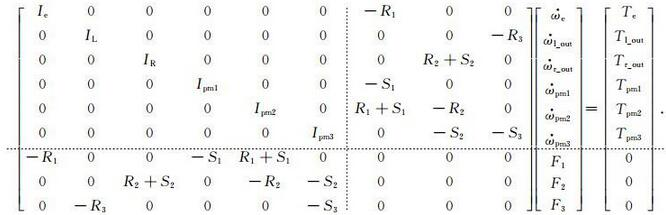(1)

R1R2R3S1S2S3分别为第1、第2、第3排行星齿轮的内齿圈半径及太阳轮半径；IeILIRIpm分别为发动机、左输出轴、右输出轴及液压泵/马达的转动惯量，由于相比于动力部件的转动惯量，行星排自身的转动惯量较小，因此可忽略不计。${\dot \omega _{\rm{e}}} $${\dot \omega _{{\rm{l\_out}}}}$$ {\dot \omega _{{\rm{r\_out}}}}$${\dot \omega _{{\rm{pm}}}}$分别为发动机、左输出轴、右输出轴、液压泵/马达的角加速度, TeTl_outTr_outTpm分别为发动机、左输出轴、右输出轴、液压泵/马达的力矩，F1F2F3为齿轮结构间的内力。

 $\begin{array}{l} \;\;\;\;\;\left[ {\begin{array}{*{20}{c}} \mathit{\boldsymbol{J}}&\mathit{\boldsymbol{D}}\\ {{\mathit{\boldsymbol{D}}^{\rm{T}}}}&{\bf{0}} \end{array}} \right]\left[ {\begin{array}{*{20}{c}} {\mathit{\boldsymbol{ \boldsymbol{\dot \varOmega} }}}\\ \mathit{\boldsymbol{F}} \end{array}} \right] = \left[ {\begin{array}{*{20}{c}} \mathit{\boldsymbol{T}}\\ {\bf{0}} \end{array}} \right],\\ \mathit{\boldsymbol{D}} = \left[ {\begin{array}{*{20}{c}} {{R_1}}&0&0\\ 0&0&{{R_3}}\\ 0&{{R_2} + {S_2}}&0\\ { - {S_1}}&0&0\\ {{R_1} + {S_1}}&{ - {R_2}}&0\\ 0&{ - {S_2}}&{ - {S_3}} \end{array}} \right]. \end{array}$ (2)

2.1.2 第二动力学特征矩阵的建立

 $\left[{\begin{array}{*{20}{c}} {{{\dot \omega }_{\rm{e}}}}\\ {{{\dot \omega }_{{\rm{l\_out}}}}}\\ {{{\dot \omega }_{{\rm{r\_out}}}}}\\ {{{\dot \omega }_{{\rm{pm1}}}}}\\ {{{\dot \omega }_{{\rm{pm2}}}}}\\ {{{\dot \omega }_{{\rm{pm3}}}}} \end{array}} \right] = {\boldsymbol{A}^*}\left[{\begin{array}{*{20}{c}} {{T_{\rm{e}}}}\\ {{T_{{\rm{l\_out}}}}}\\ {{T_{{\rm{r\_out}}}}}\\ {{T_{{\rm{pm1}}}}}\\ {{T_{{\rm{pm2}}}}}\\ {{T_{{\rm{pm3}}}}} \end{array}} \right].$ (3)

 ${\left[{\begin{array}{*{20}{c}} \mathit{\boldsymbol{J}}&\mathit{\boldsymbol{D}}\\ {{\mathit{\boldsymbol{D}}^{\rm{T}}}}&{\bf{0}} \end{array}} \right]^{ - 1}} = \left[{\begin{array}{*{20}{c}} \mathit{\boldsymbol{A}}&\mathit{\boldsymbol{B}}\\ {{\mathit{\boldsymbol{B}}^{\rm{T}}}}&\mathit{\boldsymbol{C}} \end{array}} \right],$ (4)

 $\left[{\begin{array}{*{20}{c}} {\mathit{\boldsymbol{ \boldsymbol{\dot \varOmega} }}}\\ \mathit{\boldsymbol{F}} \end{array}} \right]\left[{\begin{array}{*{20}{c}} \mathit{\boldsymbol{A}}&\mathit{\boldsymbol{B}}\\ {{\mathit{\boldsymbol{B}}^{\rm{T}}}}&\mathit{\boldsymbol{C}} \end{array}} \right] = \left[{\begin{array}{*{20}{c}} \mathit{\boldsymbol{T}}\\ {\bf{0}} \end{array}} \right],$ (5)
 $\bf{\dot{\Omega }}=\mathit{\boldsymbol{AT}}, \ \mathit{\boldsymbol{A=}}{{\mathit{\boldsymbol{A}}}^{*}},$ (6)
 $\left[\begin{matrix} \mathit{\boldsymbol{J}} & \mathit{\boldsymbol{D}} \\ {{\mathit{\boldsymbol{D}}}^{\rm{T}}} & \bf{0} \\ \end{matrix} \right]\left[\begin{matrix} \mathit{\boldsymbol{A}} & \mathit{\boldsymbol{B}} \\ {{\mathit{\boldsymbol{B}}}^{\rm{T}}} & \mathit{\boldsymbol{C}} \\ \end{matrix} \right]=\mathit{\boldsymbol{I}},$ (7)
 $\mathit{\boldsymbol{B=}}-{{\mathit{\boldsymbol{J}}}^{-\rm{T}}}\mathit{\boldsymbol{D}}{{\mathit{\boldsymbol{C}}}^{\rm{T}}},$ (8)
 ${{\mathit{\boldsymbol{C}}}^{\rm{T}}}=-{{\left( {{\mathit{\boldsymbol{D}}}^{\rm{T}}}\mathit{\boldsymbol{D}} \right)}^{-1}}{{\mathit{\boldsymbol{D}}}^{\rm{T}}}{{\mathit{\boldsymbol{J}}}^{\rm{T}}}{{\left( \mathit{\boldsymbol{D}}{{\mathit{\boldsymbol{D}}}^{\rm{T}}} \right)}^{-1}}\mathit{\boldsymbol{D}}\rm{.}$ (9)

 ${{\mathit{\boldsymbol{A}}}^{*}}=\left[\mathit{\boldsymbol{I}}-{{\mathit{\boldsymbol{J}}}^{-\rm{T}}}\mathit{\boldsymbol{D}}{{\left( {{\mathit{\boldsymbol{D}}}^{\rm{T}}}{{\mathit{\boldsymbol{J}}}^{-1}}\mathit{\boldsymbol{D}} \right)}^{-\rm{T}}}{{\mathit{\boldsymbol{D}}}^{\rm{T}}} \right]{{\mathit{\boldsymbol{J}}}^{-1}}.$ (10)

A*为一个6×6的矩阵，该动力学特征矩阵可用于自动判断相应的筛选条件。

2.2 物理可行性筛选

 ${\rm{A}}_6^3 \cdot {\rm{A}}_6^3 \cdot {\rm{A}}_6^3/3 \cdot 2 + {\rm{A}}_6^3 \cdot {\rm{A}}_6^3 \cdot {\rm{C}}_3^2{\rm{A}}_6^2/2 = 1\;800\;000.$ (11)

1) 3个电机构成同一排行星齿轮；

2) 两个输出在同一排行星齿轮，剩余节点与发动机或制动器相连(两侧转速相互耦合，无法实现独立控制)；

3) 发动机与其中一个输出在同一排行星齿轮中，且该排余下节点与制动器相连(输出转速与发动机转速相互耦合)；

4) 不可缺少任意一个系统部件。

2.3 系统特性可行性筛选

 特性 条件 双输出独立控制及转向 ${\rm{rank}}\left[{\begin{array}{*{20}{c}} {a_{21}^*}&{a_{22}^*}&{a_{23}^*}&{a_{24}^*}&{a_{25}^*}&{a_{26}^*}\\ {a_{31}^*}&{a_{32}^*}&{a_{33}^*}&{a_{34}^*}&{a_{35}^*}&{a_{36}^*} \end{array}} \right] = 2,$ a36*=2, a21*>0, a31*>0 倒退功能 a21* < 0, a31* < 0;或a24* < 0, a34* < 0;或a25* < 0, a35* < 0;或a26* < 0, a36* < 0 中心转向功能 a21*·a31* < 0;或a24*·a34* < 0;或a25*·a35* < 0;或a26*·a36* < 0

2.4 综合行驶性能可行性筛选

2.5 基于动态规划的燃油经济性分析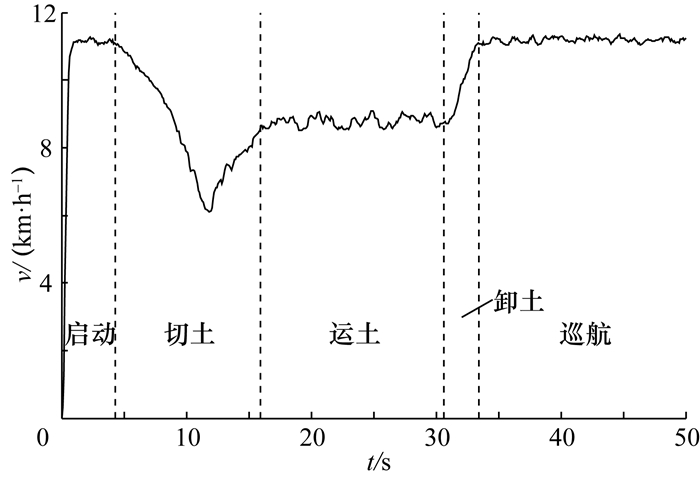图 4 履带推土机实际循环工况示意图

 $J = \sum\limits_{k = 0}^{N - 1} {L\left( {x\left( k \right),u\left( k \right)} \right) + \alpha {{\left( {{\rm{SOC}}\left( N \right) - {\rm{SOC}}\left( 0 \right)} \right)}^2}.}$ (12)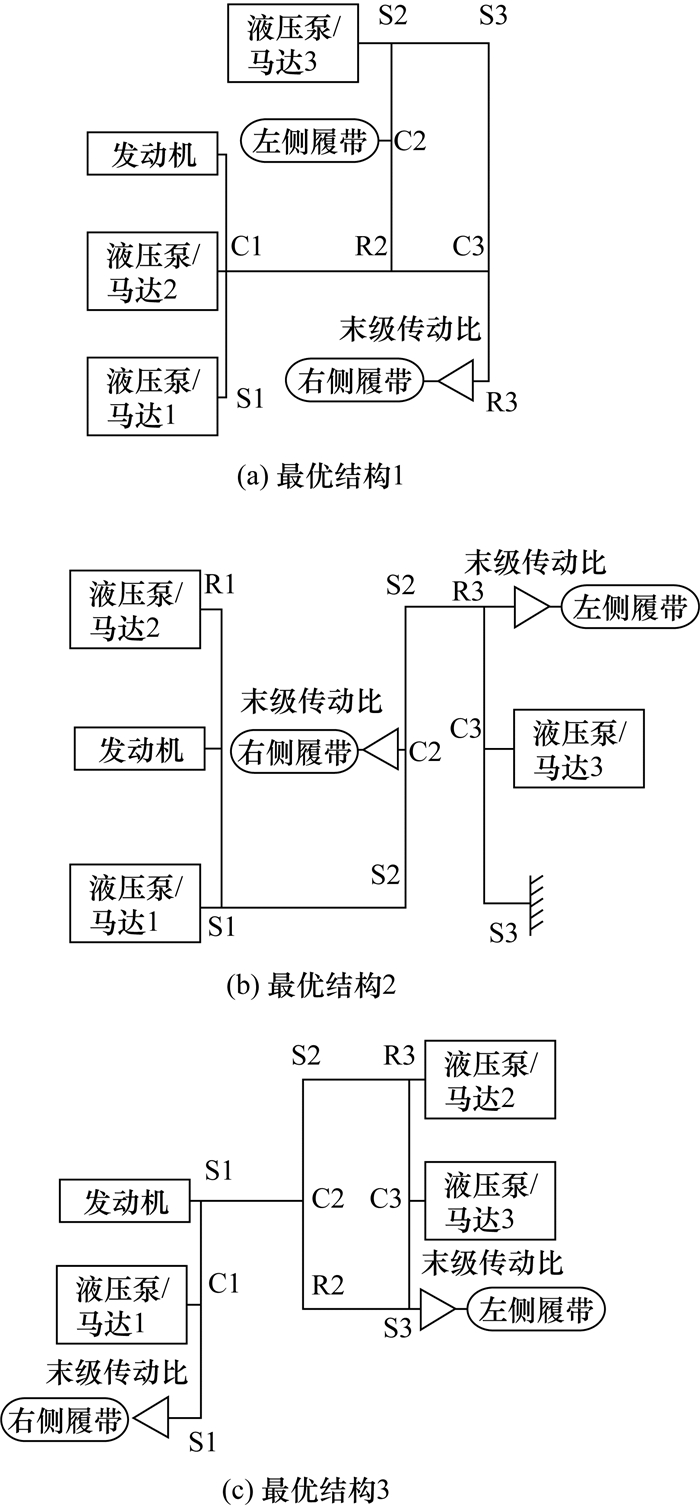图 5 最终筛选得到的最优设计方案

3 最优结构的仿真结果分析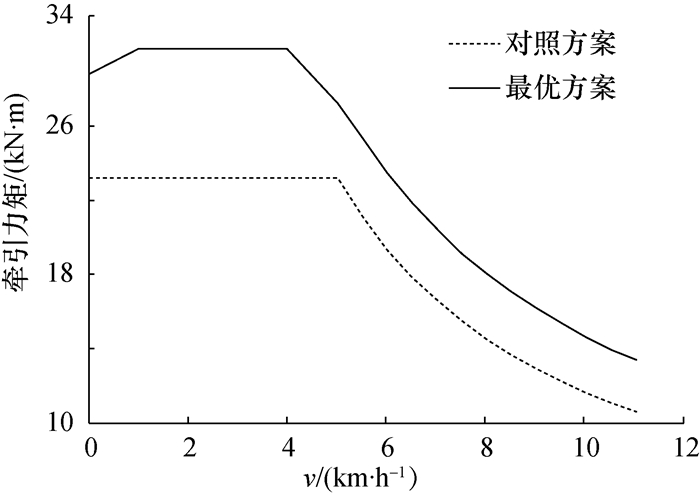图 6 直线行驶性能对比分析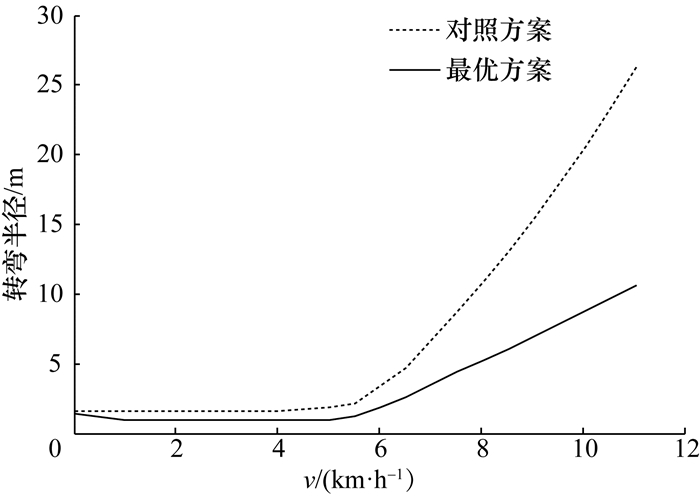图 7 转向行驶性能对比分析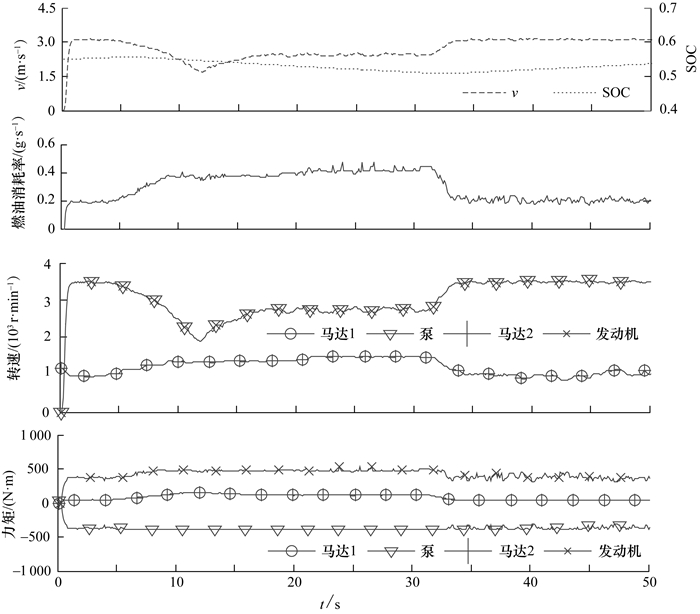图 8 对照方案动态规划仿真结果图 9 最优方案的动态规划仿真结果

4 结论

  Xiao Q, Wang Q, Zhang Y. Control strategies of power system in hybrid hydraulic excavator[J]. Automation in Construction, 2008, 17(4): 361–367. DOI:10.1016/j.autcon.2007.05.014  Jo D Y, Kwak S, Kim N. Development of fuel-efficient construction equipment [C]//2011 IEEE 8th International Conference on Power Electronics and ECCE Asia (ICPE & ECCE). Jeju, Korea, 2011: 31-37.  马鹏飞. 全液压推土机液压行驶驱动系统动力学研究[D]. 西安: 长安大学, 2006. MA Pengfei. Dynamics Research of the Hydraulic Bulldozer Driving System [D]. Xi'an: Changan University, 2006. (in Chinese)  ZHAO Yong, ZHOU Lin. Parameter matching theory of hybrid track-type bulldozer power system [C]//World Automation Congress. Puerto Vallarta, Mexico, 2012: 24-28.  Surampudi B, Nedungadi A, Ostrowski G, et al. Design and Control Considerations for a Series Heavy Duty Hybrid Hydraulic Vehicle [R]. SAE Technical Paper 2009-01-2717, 2009.  Meyers R A. Encyclopedia of Sustainability Science and Technology[M]. New York, NY, USA: Springer Science & Business Media, 2012.  邹渊, 陈晓玲, 李东阁, 等. 串联式混合动力推土机驱动系参数匹配设计[J]. 机械工程学报, 2014, 50(1): 70–75. ZOU Yuan, CHEN Xiaoling, LI Dongge, et al. Parameter matching design and control optimization for series hybrid tracked bulldozer[J]. Journal of Mechanical Engineering, 2014, 50(1): 70–75. (in Chinese)  Liu J, Peng H. Modeling and control of a power-split hybrid vehicle[J]. IEEE Transactions on Control Systems Technology, 2008, 16(6): 1242–1251. DOI:10.1109/TCST.2008.919447  Cho S, Ahn K, Lee J M. Efficiency of the planetary gear hybrid powertrain[J]. Proceedings of the Institution of Mechanical Engineers, Part D: Journal of Automobile Engineering, 2006, 220(10): 1445–1454. DOI:10.1243/09544070JAUTO176  Kahraman A. Planetary gear train dynamics[J]. Journal of Mechanical Design, 1994, 116(3): 713–720. DOI:10.1115/1.2919441  Li C T, Peng H. Optimal configuration design for hydraulic split hybrid vehicles [C]//Proceedings of the 2010 American Control Conference. Baltimore, MD, USA, 2010: 5812-5817.  Zhuang W, Zhang X, Ding Y, et al. Comparison of multi-mode hybrid powertrains with multiple planetary gears[J]. Applied Energy, 2016, 178: 624–632. DOI:10.1016/j.apenergy.2016.06.111  Zhang X, Peng H, Sun J. A near-optimal power management strategy for rapid component sizing of multimode power split hybrid vehicles[J]. IEEE Transactions on Control Systems Technology, 2015, 23(2): 609–618. DOI:10.1109/TCST.2014.2335060